Notes on spherical trigonometry

(by Roberto Bigoni)

1. Spheres, geodesics, lunes.

A circumference, obtained by intersecting a spherical surface Σ of radius R with a plane passing through its center, ie one of the circumferences of maximum radius obtainable on the spherical surface, is called a geodesic. The secant plane is called the generating plane of the geodesic.

For example, assuming that the Earth is a perfect sphere, all the meridians are geodesics, but the only parallel that is a geodesic is the equator.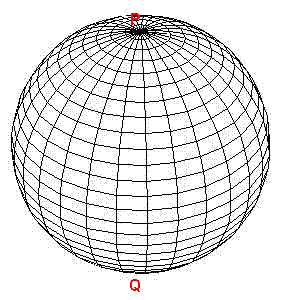Two different geodesics intersect in two diametrically opposed points P and Q. Their generating planes form two pairs of vertically opposite dihedrals. Each dihedral, with measure λ, forms on spherical surface a region, bounded by two semicircles with extremes P and Q, said a lune, with vertices P and Q and sides PAQ and PBQ. The points P and Q are the vertices of two spherical angles, both measuring λ. We can also say that the lune PAQB is the lune Λ with angle λ.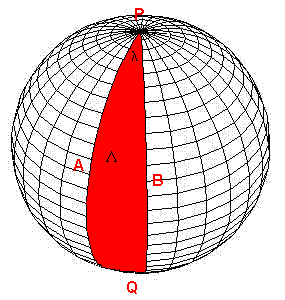The ratio between the area l of the lune Λ with angle λ and the surface Σ is equal to the ratio between the angle λ and .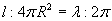then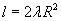The set of all the geodesics, which intersect at the same points P and Q, is said pencil of geodesics with poles PQ.

For example, two terrestrial meridians intersect in the geographic poles N and S.

The set of all the meridians is a pencil of geodesics with poles NS.

2. Spherical triangles.

If we intersect a lune Λα with angle α of a pencil with poles AZ, with a third geodesic, which does not belong to the same pencil, and that therefore intersects the sides of Λα at points B and C, we divide the lune Λα in two regions with vertices ABC and ZBC, called spherical triangles.

The area of the triangle ZBC is obtained by subtracting the area of the triangle ABC from the area of the lune Λα.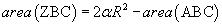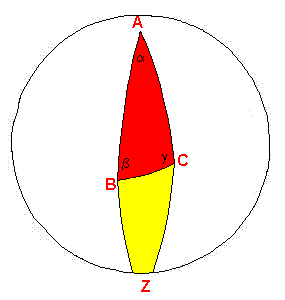The triangle ABC is the intersection of the lune Λβ, with angle β, and Λγ with angle γ. So the area of the union of Λβ and Λγ is given by the sum of their areas decreased by the area of the triangle ABC (which, otherwise, would be evaluated twice).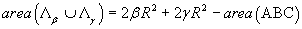Furthermore, if we join the triangle with vertices at points diametrically opposite to Z, B and C, which is congruent with the triangle ZBC, with the union of the lunes Λβ and Λγ, we obtain a hemisphere. So, if we we add the area of the triangle ZBC with the area of the union of Λβ and Λγ, we obtain the surface area of the hemisphere.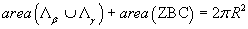Combining these results, we obtain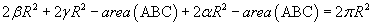so the area of the spherical triangle ABC is: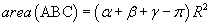Since the area of a spherical triangle must be positive, we infer that the sum of the internal angles of a spherical triangle is greater than a straight angle.

This result is evident in the pencil of the terrestrial meridians: both two different meridians form straight angles with the equator and, converging in a pole, form an angle given by the absolute value of the difference between their longitudes. So on the terrestrial surface, in a spherical triangle delimited by two different meridians and by the arc of equator between them, the sum of the internal angles is greater than two right angles.

3. Euler's formula.

Let us consider a convex polyhedron Π with triangular faces and let F be the number of its faces, V the number of its vertices and S the number of its edges. Let Σ be a spherical surface, with radius R, containing the polyhedron Π and from a point P, placed within Π, let us project the edges of Π itself on Σ. For each of the faces of Π there will be a corresponding spherical triangle, for each of the edges of Π the will be a corresponding side of a triangle and for each vertex of Π there will be a corresponding point, in which the sides of the contiguous triangles converge.

The area of each spherical triangle is given by the product of the difference between the sum of its interior angles and a straight angle, multiplied by the square of the radius.

The area of the spherical surface is equal to the sums of the areas of all the triangles, that is, the sum of all the angles of triangles decreased by as many straight angles as faces.

The sum of the interior angles is equal to as many full angles as vertices.

Therefore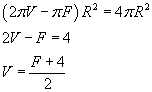Since each side is common to two contiguous triangles, the number S of sides is equal to half of three times the number F of faces: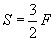Therefore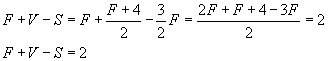If Π has polygonal faces (therefore not necessarily triangular), its projection on Σ would be a grid formed by spherical polygons, which, if they had more than three sides, may be divided into triangles, by tracing their diagonals. For each plotted diagonal, we have an increase by one unit in both the number of sides and the number of faces, and the relation between the numbers of faces, edges and vertices remains unchanged. So in general we obtain that
in every polyhedron, the sum of the number of faces and vertices exceeds by two the number of edges.

This result is one of the most famous among those obtained by L. Euler.

4. The Pythagoras' theorem.

Let us consider, on a spherical surface Σ with radius R and center O, a spherical triangle with vertices A, B and C, such that the internal angle ACB is a right angle. Let a, b and c be the measures of the sides respectively opposite to A, B and C.

In this case we can choose an orthogonal Cartesian reference system with origin in the center O, such that the plane Oyz coincides with the generating plane of the side AC and the plane Oxz coincides with the generating plane of the side BC.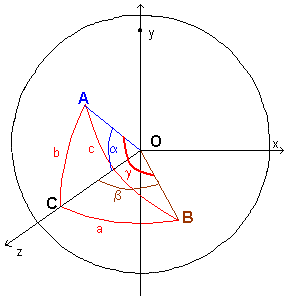We can describe the segments OA and OB as vectors of length R. Let α be the measure of the angle AOC, β the measure of the angle BOC and i, j and k be the unit vectors of the cartesian axes. Then: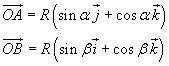The scalar product of the two vectors is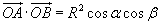We can write the scalar product as the product of their magnitudes by the cosine of the angle AOB with measure γ: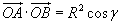So we have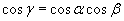Since the measure in radians of an angle at the center of a circumference is given by the ratio between the arc and the radius,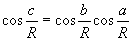This result is a generalization of the Pythagoras' theorem. In fact, from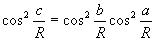we get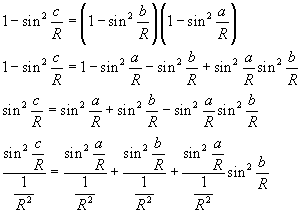If we increase to infinity the spherical surface so that a limited region on it becomes indistinguishable from a flat region, we must calculate the limit of this equality as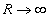. We get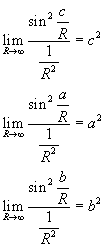but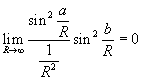So to the limit we have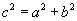5. Length of an arc.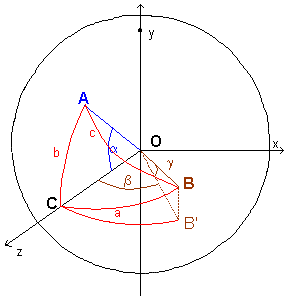Let A and B be two points on a spherical surface Σ with radius R. Let C be the point of the equator belonging to the meridian of A. If we assume the longitude of A as 0, the vectors OA and OB are can be expressed as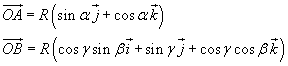The scalar product of the two vectors is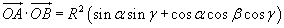and can be written also as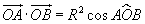then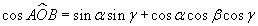The length of the arc AB is given by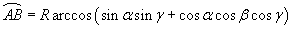In particular, if A and B are points on the surface of the Earth and if we assume the Earth as a perfect sphere, we have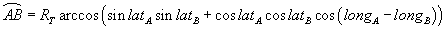Example: calculation of the distance (as the crow flies) between New York and Cape Town.

placelatitudelongitude
New York40°45'25"N73°59'10"W
Cape Town33°54'58"S18°25'19"E

We decimalize the measures of the geographical coordinates.

placelatitudelongitude
New York40.757140°-73.986053°
Cape Town-33.916156°18.422012°

placelatitudelongitude
New York0.711346-1.291300
Cape Town-0.5919490.321525

We calculate the difference of longitude::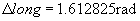and the measure of the angle γ: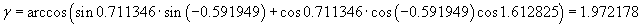Finally, the distance between New York and Cape Town is obtained by multiplying the mean Earth's radius by γ: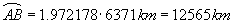Measurements obtained with more elaborate calculations, based on the fact that the Earth is not a perfect sphere but an ellipsoid, give a distance of 12552km.
As you can see the error is of the order of 1 ‰.

Here the distance between two points on a perfect spherical surface is calculated by Javascript. Avoid pairs of diametrically opposite points.
If your browser does not admit the iframe tag, you can directly open the application page

6. The cosine theorem.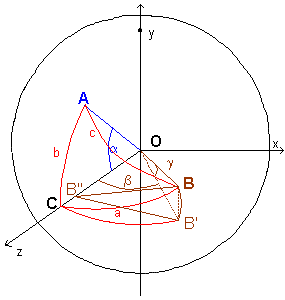Let A, B and C be three points on a spherical surface with unit radius. We have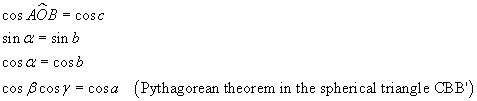Moreover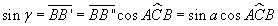If we substitute the right hand sides of this identity in the equationwe get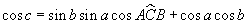This identity expresses c as a function of the cosine of the angle formed by the two other sides. So it is the spherical version of the well-known theorem of the cosine of plane trigonometry. If the angle ACB is right we get the theorem of Pythagoras.

From the measurements of the sides we obtain the cosines of the interior angles of the triangle: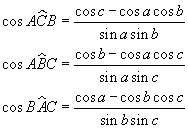If a sphere has radius R we have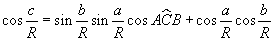then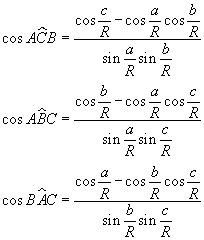7. Perimeter and area of a triangle.Given a triangle ABC on a spherical surface Σ with unit radius and center O, if we know the angles α, β e γ in the above figure (that is latitudes and longitudes of A, B and C with respect to a suitable reference system on Σ), if a, b and c are the measures of the the sides, the measures of the interior angles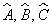can be deduced from the measures of a, b and c by using the cosine theorem. The area of the triangle, as we have seen in Section 2, is obtained by subtracting π from the sum of the interior angles. The measure of this area coincides with the measure in steradians of solid angle with vertex in O and sides determined by the intersections of the generating half-planes of the sides of the triangle.

If Σ has radius R, the steradians must be multiplied by R2.

To solve a spherical triangle, it is useful to implement the calculation in a spreadsheet or in a sequential programming language. Here we propose a Javascript version. Latitudes and longitudes are written as sexagesimal degrees (ggg°mm'ss" with at least one of the three separators) or as radians (a pure decimal). Avoid pairs of diametrically opposite points.
If your browser does not admit the iframe tag, you can directly open the application page

8. The law of sines.

Let A, B and C be the vertexes of a triangle on a spherical surface Σ with center O and radius R. Let us draw the radii from O to the vertexes and let α, β and γ be the angles between them. Let us consider the plane from C perpendicular to the plane of the radii OA and OB and let A' and B' be the intersections of this plane with the two radii. Let us consider the triangle CA'B' and let CH be the height of this triangle with respect to the side A'B'. Let h be the measure of CH.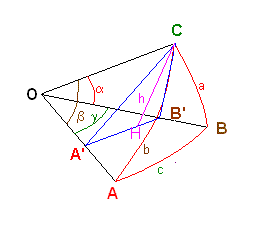By applying well known formulas of plane trigonometry, we get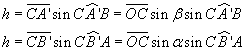then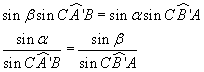The angles at the denominators coincide with the angles at the vertexes of spherical triangle ABC; those at the numerators are given by the ratios between the sides of the ABC and the radius R. Therefore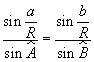The same construction can be repeated on each of the vertexes of the triangle, so in general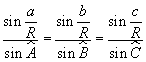If, this relation converges to the law of sines of plane trigonometry.

last revision: November 2018